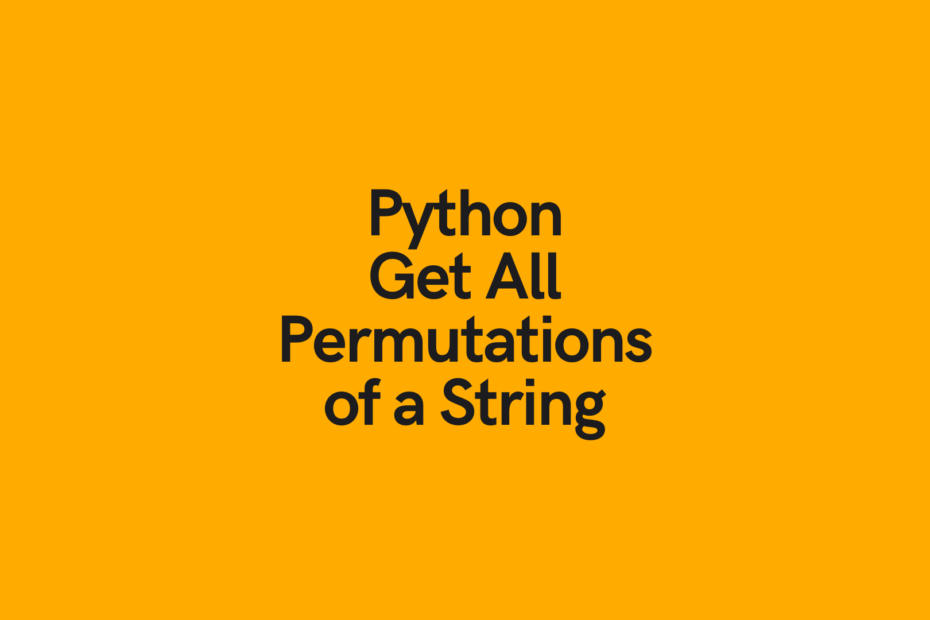# Python: Find All Permutations of a String (3 Easy Ways!)In this tutorial, you’ll learn how to use Python to find all permutations of a string, including using `itertools`, recursion, and Python for loops. You will also learn how to find all combinations of a string when replacement of individual letters are allowed.

But what is a permutation? A permutation is a different ordering of an item. So, for example, the string `abc` can also be written as `['abc', 'acb', 'bac', 'bca', 'cab', 'cba']`.

The Quick Answer: User Itertools to Find All Permutations of a String

## What are Permutations of a String?

Permutations of a string refers to all the different orderings a string may take. Let’s, for example, take a look at a string that takes up three letters: `'abc'`. When we find all the permutations of this string, we return the following list: `['abc', 'acb', 'bac', 'bca', 'cab', 'cba']`. We can see here, that we have a list that contains six items.

We can actually calculate the number of permutations a string will have for any length strength, by calculating the factorial of its length. So, in our example of `'abc'`, we would calculate the value of `3!`, which actually evaluates to `3x2x1 = 6`.

Now that you have an understanding of what it means to generate all combinations of a string in Python, let’s see how we can easily generate the different permutations of a string.

## Use Itertools in Python to Find All Permutations of a String

Itertools is a fantastic, built-in Python tool that allows you to make easy work of problems involving iterables. Believe it or not, strings in Python are iterable objects! Because of this, we can easily iterate over our strings using the `itertools` library.

In fact, the itertools library has a function called `permutations`. We we pass in an iterable, in this case, a string, the function returns a list of all possible combinations.

Let’s take a look at our example string and how we can use the itertools library to calculate its permutations:

``````import itertools

a_string = 'abc'
string_permutations = itertools.permutations(a_string)
string_permutations = list(string_permutations)
string_permutations = [''.join(permutation) for permutation in string_permutations]

print(string_permutations)

# Returns: ['abc', 'acb', 'bac', 'bca', 'cab', 'cba']``````

Let’s take a look at what we’ve done here:

1. We imported the itertools library
2. We loaded our string and assigned it to the variable `a_string`
3. We then used the `permutations()` function to create a itertools object
4. We turned this object into a list, which returned a list of tuples, containing our permutations
5. Finally, we used a list comprehension to combine our permutations into individual strings

In the next section, you’ll learn how to use recursion to find combinations of a string in Python.

Want to learn more about Python list comprehensions? Check out this in-depth tutorial that covers off everything you need to know, with hands-on examples. More of a visual learner, check out my YouTube tutorial here.

## Use Recursion in Python to Find All Permutations of a String

The concept we’ll use in recursion to create permutations is known as backtracking. The idea is that we backtrack for each possible combination that can exist.

Let’s take a look at how this recursive method works to help find all combinations of a string in Python:

``````# Getting all permutations of a string using recursion in Python
a_string = 'abc'

def get_permutation(some_string, idx=0):

if idx == len(some_string) - 1:
print("".join(some_string))

for j in range(idx, len(some_string)):
words_list = [c for c in some_string]
words_list[idx], words_list[j] = words_list[j], words_list[idx]

get_permutation(words_list, idx + 1)

permutations = get_permutation(a_string)
print(permutations)

# Returns: ['abc', 'acb', 'bac', 'bca', 'cab', 'cba']``````

We can see that this returns the same as above. However, it is a bit less intuitive to follow along for beginner Python users, so perhaps using the itertools method might be preferred for readability. This method, however, is helpful for when you cannot use itertools for some reason.

Want to learn how to use the Python `zip()` function to iterate over two lists? This tutorial teaches you exactly what the `zip()` function does and shows you some creative ways to use the function.

## Permutations with Repetition of a String in Python

In the examples above, we used Python to find all combinations of a string without repetition. In this section, you’ll learn how to use Python to get all permutations of a string with repetition.

This can be easily done using a Python for loop.

Let’s take a look at an example, using the same string that we have used before, `'abc'`:

``````# Use Python to get all combinations of a string with repetition
a_string = 'abc'

final_list = [[]]
length = len(a_string)
groups = [list(a_string)] * length
for i in groups:
final_list = [x+[y] for x in final_list for y in i]

permutations = [''.join(item) for item in final_list]
print(permutations)

# Returns ['aaa', 'aab', 'aac', 'aba', 'abb', 'abc', 'aca', 'acb', 'acc', 'baa', 'bab', 'bac', 'bba', 'bbb', 'bbc', 'bca', 'bcb', 'bcc', 'caa', 'cab', 'cac', 'cba', 'cbb', 'cbc', 'cca', 'ccb', 'ccc']``````

In the code above, we use a for loop and a list comprehension to return all combinations of a Python string with repetition.

The code above can become quite resource intensive. We can also see here that we have many times more combinations when we use repetition than if we do not.

In this post, you learned how to use Python to generate a list of all permutations of a string. You learned how to do this using the popular `itertools` library as well as using recursion. You then learned how to use a Python for loop to generate a list of all combinations of a string when repetition is allowed.
To learn more about the `permutation()` function, check out the official documentation.